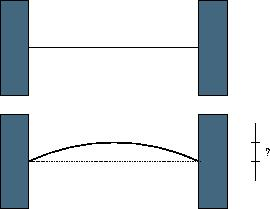# Competitive Programming: POJ 1905 – Expanding Rods

### DescriptionWhen a thin rod of length L is heated n degrees, it expands to a new length L’=(1+n*C)*L, where C is the coefficient of heat expansion.

When a thin rod is mounted on two solid walls and then heated, it expands and takes the shape of a circular segment, the original rod being the chord of the segment.

Your task is to compute the distance by which the center of the rod is displaced.

### Input

The input contains multiple lines. Each line of input contains three non-negative numbers: the initial lenth of the rod in millimeters, the temperature change in degrees and the coefficient of heat expansion of the material. Input data guarantee that no rod expands by more than one half of its original length. The last line of input contains three negative numbers and it should not be processed.

### Output

For each line of input, output one line with the displacement of the center of the rod in millimeters with 3 digits of precision.

### Sample Input

1000 100 0.0001
15000 10 0.00006
10 0 0.001
-1 -1 -1


### Sample Output

61.329
225.020
0.000


Solution below . . .

### SolutionWe need a couple of equations: One is how to calculate an arc length knowing its subtended chord and the radius of the circle (see figure). The chord is analogous to the original rod and the arc to the heated rod.

Here’s the equation for the arc length where the angle returned by$asin$ is in radians:$arc = 2r \cdot asin(AB/2r)$     (1)

Derivation: (feel free to skip it if it’s obvious or you’re not interested)

1.$sin(BCD) = (AB/2)/r$
2. Central angle that intercepts arc$= 2 cdot asin(AB/2r)$
3.$arc = r \cdot angle = 2r \cdot asin(AB/2r)$

The variable we want to calculate is$s$, which is called the sagitta. If we could find the sagitta length directly from the arc length and the chord length, both of which we know, then our task would be very easy, but I don’t know a way to do that and I don’t think anyone else does either.

Instead, let’s write down an equation for the radius$r$. If we could determine$s$ then, since triangle$DCB$ is a right triangle, Pythagoras tells us$(c/2)^2 + (r - s)^2 = r^2$

Solving for$r$ gives$r = (4s^2 + c^2)/(8s)$     (2)

We can’t solve that directly because we don’t know$s$, but we can use binary search to get successively better approximations of$s$.

After each approximation, we plug the result of (2) into (1) until we’re within an acceptable tolerance.

import java.util.Scanner;

public class Main {

static final double EPS = 1e-5;

public static void main(String[] args) {

Scanner sc = new Scanner(System.in);

while (sc.hasNext()) {
double L = sc.nextDouble();
double n = sc.nextDouble();
double C = sc.nextDouble();

if (L < 0 && n < 0 && C < 0)
break;

double LL = (1 + n * C) * L;
double low = 0;
double high = L / 2;

double R, mid = 0;

while (high - low > EPS) {
mid = (high + low) / 2;
R = ((mid * mid * 4) + (L * L)) / (8 * mid);
if ((2 * R * Math.asin(L / (2 * R))) < LL)
low = mid;
else
high = mid;
}
System.out.printf("%.3fn", mid);
}
}
}